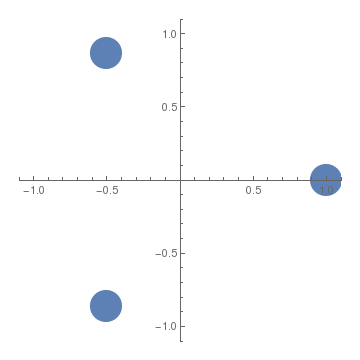# Preface

Complex numbers were introduced by the Italian famous gambler and mathematician Gerolamo Cardano (1501--1576) in 1545 while he found the explicit formula for all three roots of a cube equation. Many mathematicians contributed to the full development of complex numbers. The rules for addition, subtraction, multiplication, and division of complex numbers were developed by the Italian mathematician Rafael Bombelli (baptized on 20 January 1526; died 1572). The notations 1 and i for unit vectors in horizontal positive direction and vertical positive direction, respectively, were introduced by Leonhard Euler (1707--1783) who visualized complex numbers as points with rectangular coordinates, but did not give a satisfactory foundation for complex numbers theory. He also suggested to drop the unit vector 1 in presenting vectors on the plane. It was Carl Friedrich Gauss (1777--1855) who introduced the term complex number. Cauchy, a French contemporary of Gauss, extended the concept of complex numbers to the notion of complex functions. Professor Orlando Merino (born in 1954) from the University of Rhode Island has written an essay on the history of the discovery of complex numbers.

# Complex numbers

Complex numbers can be identified with three sets: the set of points on the plane (denoted by ℝ²), set of all (free) vectors on the plane, and the set of all ordered pairs of real numbers z = (x,y). In the latter set, the first coordinate of z = (x,y) is denoted by ℜz = x (or Rez) and is called, for historical reasons, the real part of complex number z, while the second coordinate is denoted by ℑ ℜz = y (or Imzz. A geometric plot of complex numbers as points z = x + jy using the x-axis as the real axis and y-axis as the imaginary axis is referred to as an Argand diagram. This geometric plot is named after Jean-Robert Argand (1768–1822), who introduced it in 1806, although it was first described by Norwegian–Danish land surveyor and mathematician Caspar Wessel (1745–1818).

The set of complex numbers is one of the three sets above equipped with arithmetic operations (addition, subtraction, multiplication, and division) that satisfy the usual axioms of real numbers. While addition and subtraction are inherited from vector algebra, multiplication and division satisfy specific rules based on the identity j² = -1.

For a long time it was thought that complex numbers were just toys invented and played with only by mathematicians. After all, no single quantity in the real world can be measured by an imaginary number, a number that lives only in the imagination of mathematicians. However, in 1926, Erwin Schrödinger (1887--1961) discovered that in the language of the world of the subatomic particles, complex numbers were the indispensable alphabet. Although no single measurable physical quantity corresponds to a complex number, a pair of physical quantities can be represented very naturally by a complex number. For instance, a wave, which always consists of an amplitude and a phase, begs a representation by a complex number.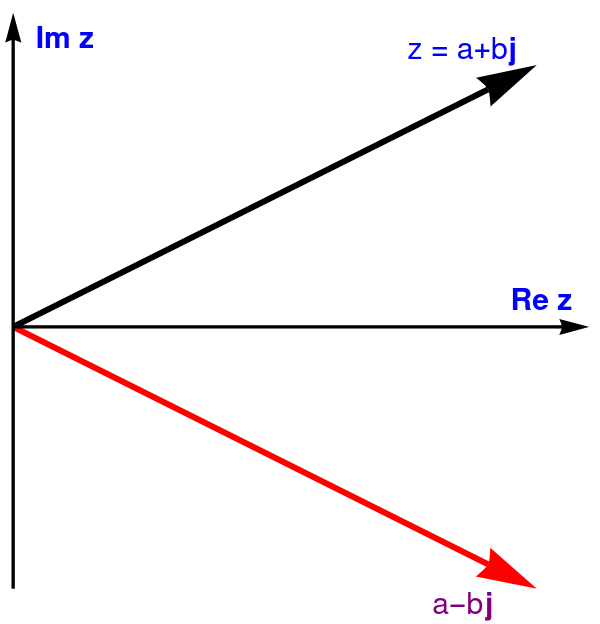vector = Graphics[{Black, Arrowheads[0.1], Thickness[0.01], Arrow[{{0, 0}, {2, 1}}]}]; conjugate = Graphics[{Red, Arrowheads[0.1], Thickness[0.01], Arrow[{{0, 0}, {2, -1}}]}]; ax = Graphics[{Black, Arrowheads[0.05], Thick, Arrow[{{0, 0}, {2.2, 0}}]}]; ay = Graphics[{Black, Arrowheads[0.05], Thick, Arrow[{{0, -1}, {0, 1.2}}]}]; txtj = Graphics[ Text[Style["j", Bold, FontSize -> 18, Blue], {1.91, 1.06}]]; txtC = Graphics[ Text[Style["z = a+b", FontSize -> 18, Blue], {1.7, 1.06}]]; txtjc = Graphics[ Text[Style["j", Bold, FontSize -> 18, Purple], {1.82, -1.06}]]; txtCC = Graphics[ Text[Style["a-b", FontSize -> 18, Purple], {1.7, -1.06}]]; tx = Graphics[ Text[Style["Re z", Bold, FontSize -> 18, Blue], {2.02, 0.1}]]; ty = Graphics[ Text[Style["Im z", Bold, FontSize -> 18, Blue], {0.2, 1.1}]]; Show[vector, conjugate, ax, ay, txtj, txtC, txtCC, txtjc, tx, ty] Complex number z and its conjugate. Mathematica code

It is a customary to visualize a complex number as a vector, denoting its horizontal component by Re (real part) and its vertical component by Im (imaginary part). In Cartesian coordinates, a complex number is denoted by the ordered pair:
$z = (a, b) , \qquad\mbox{with} \qquad \Re z = \mbox{Re}z = a, \quad \Im z = \mbox{Im}z = b .$
It was Leonhard Euler who suggested to use two unit vectors, 1 for the abscissa and i for the ordinate, allowing him to write
$z = (a, b) = a\,1 + b\,i = a + b\,i ,$
upon dropping multiple 1. Mathematicians still follow this genius. However, in physics, engineering, and computer science, unit vectors are usually denoted by i, j, and k (most likely, you met them in calculus). Then on the plane, a complex number can be written as
$z = (a, b) = a + b\,{\bf j} = a + {\bf j}\,b ,\qquad\mbox{with} \qquad \Re z = \mbox{Re}z = a, \quad \Im z = \mbox{Im}z = b .$
In what follows, we use j as a unit vector in the positive vertical direction and call it the imaginary unit. A complex number can also be written in polar form
$z = (a, b) = a + b\,{\bf j} = r\,e^{{\bf j}\theta} , \qquad r = \sqrt{x^2 + b^2}.$
Angle θ is measured in counterclockwise direction from the real axis. The complex form is based on Euler's formula:
\begin{equation} \label{EqComplex.1} e^{{\bf j} \theta} = \cos\theta + {\bf j}\,\sin\theta . \end{equation}
Given the complex number z = 𝑎 + bj, its complex conjugate, denoted either with an overline (in mathematics) or with an asterisk (in physics and engineering), is the complex number reflected across the real axis:
$z^{\ast} = (a + b\,{\bf j})^{\ast} = \overline{z} = \overline{a + b\,{\bf j}} = a - b\,{\bf j} = r\, e^{-{\bf j}\theta} , \qquad \mbox{for}\quad z = r\,e^{{\bf j}\theta} .$

Now we are ready to define the field of complex numbers, denoted by ℂ.
The field of complex numbers, denoted by ℂ, is the set of all ordered pairs of real numbers together with the following arithmetic operations.
1. Addition:       z₁ + z₂ = (x₁ + y₁ j) + (x₂ + y₂ j) = x₁ + x₂ + j (y₁ + y₂).
2. Substraction:       z₁ − z₂ = (x₁ + y₁ j) − (x₂ + y₂ j) = x₁ − x₂ + j (y₁ − y₂).
3. Multiplication:       z₁ ⋅ z₂ = (x₁ + y₁ j) ⋅ (x₂ + y₂ j) = (xx₂ − yy₂) + j (xy₂ + xy₁).
4. Division:       division of two complex numbers z₁ ⁄ z₂ = (x₁ + y₁ j) ÷ (x₂ + y₂ j) is
$\frac{x_1 + {\bf j}\,y_1}{x_2 + {\bf j}\,y_2} = \frac{x_1 x_2 + y_1 y_2}{x_2^2 + y_2^2} + {\bf j}\,\frac{x_2 y_1 - x_1 y_2}{x_2^2 + y_2^2}.$
From the multiplication axiom, it immediately follows that j² = −1.

Multiplication and division have extremely simple form when polar form is employed. Suppose we need to multiply two complex numbers

$z= r\,e^{{\bf j}\,\theta} \qquad \mbox{and} \qquad w = R\,e^{{\bf j}\,\alpha} \qquad \Longrightarrow \qquad z\cdot w = r\,R\,e^{{\bf j}\left( \theta + \alpha \right)} .$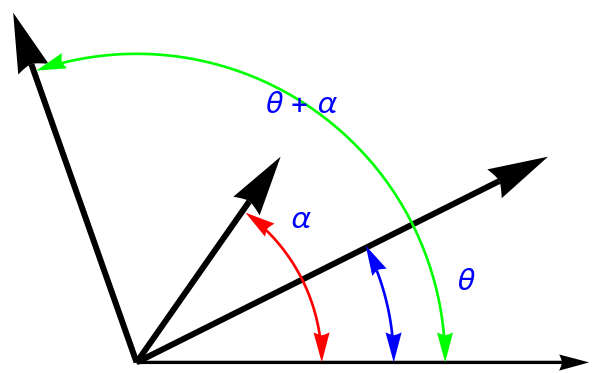vector = Graphics[{Black, Arrowheads[0.1], Thickness[0.01], Arrow[{{0, 0}, {2, 1}}]}]; vec2 = Graphics[{Black, Arrowheads[0.1], Thickness[0.01], Arrow[{{0, 0}, {0.7, 1}}]}]; vec = Graphics[{Black, Arrowheads[0.1], Thickness[0.01], Arrow[{{0, 0}, {-0.6, 1.7}}]}]; ax = Graphics[{Black, Arrowheads[0.05], Thick, Arrow[{{0, 0}, {2.2, 0}}]}]; aarr = Show[ ParametricPlot[#[]*{Cos[$Theta]], Sin[\[Theta]]}, {\[Theta], #[], #[]}, Axes -> False, PlotStyle -> #[]] /. Line[x_] :> Sequence[Arrowheads[{-0.05, 0.05}], Arrow[x]] & /@ {{0.9, 0 Degree, 54 Degree, Red}, {1.25, 0 Degree, 27 Degree, Blue}, {1.5, 0 Degree, 109 Degree, Green}}, PlotRange -> All] txz = Graphics[ Text[Style["\[Theta]", FontSize -> 18, Blue], {1.6, 0.4}]]; txw = Graphics[ Text[Style["\[Alpha]", FontSize -> 18, Blue], {0.8, 0.7}]]; txzw = Graphics[ Text[Style["\[Theta] + \[Alpha]", FontSize -> 18, Blue], {0.8, 1.26}]]; Show[vector, vec2, vec, ax, aarr, txz, txw, txzw] Multiplication of two complex numbers. Mathematica code This also allows us to easily find all roots of any complex number. Suppose that we want to calculate the n roots of a complex number $$z = r\,e^{{\bf j}\,\theta} = r \left( \cos\theta + {\bf j}\,\sin\theta \right) .$$ Then all n roots are determined from the formula: \[ z^{1/n} = \left( r\,e^{{\bf j}\,\theta} \right)^{1/n} = \left\{ \sqrt[n]{r} \left( \cos \frac{\theta + k\,2\pi}{n} + {\bf j}\,\sin \frac{\theta + k\,2\pi}{n} \right) \right\} , \qquad k=0,1,2,\ldots , n-1.$

Example 1: Let us consider the complex number 4 + 3 j that we represent in polar form

$4 + 3\,{\bf j} = 5\,e^{{\bf j}\,\theta} , \qquad \mbox{where}\quad \theta = \arctan \frac{3}{4} \approx 0.643501 .$
N[ArcTan[3/4]]
0.643501
If we want to find all three cubic roots, we need to know the angles:
N[ArcTan[3/4]/3]
0.2145
N[(2*Pi + ArcTan[3/4])/3]
2.3089
N[(4*Pi + ArcTan[3/4])/3]
4.40329
Since
N[5^(1/3)]
1.70998
we find all three cubic roots:
$\left( 4 + 3\,{\bf j} \right)^{1/3} \approx 1.70998 \left( \cos 0.2145 + {\bf j}\,\sin 0.2145 \right) =\approx 1.67079 + {\bf j}\,0.363984 .$
N[5^(1/3)]*Cos[ArcTan[3/4]/3]
1.67079
$\left( 4 + 3\,{\bf j} \right)^{1/3} \approx 1.70998 \left( \cos 2.3089 + {\bf j}\,\sin 2.3089 \right) =\approx -1.15061 + {\bf j}\, 1.26495.$
N[5^(1/3)]*Sin[(2*Pi + ArcTan[3/4])/3]
1.26495
$\left( 4 + 3\,{\bf j} \right)^{1/3} \approx 1.70998 \left( \cos 4.40329 + {\bf j}\,\sin 4.40329 \right) =\approx -0.520175 - {\bf j}\,1.62894 .$
N[5^(1/3)]*Cos[(4*Pi + ArcTan[3/4])/3]
-0.520175

# Mathematica for Complex numbers

Since there is no universal notation for a unit vector in vertical direction on the complex plane, Mathematica uses two of them: i and j. Euler suggested to use i ($${\bf i}^2 =-1$$ ) , so mathematicians follow him; however, in engineering and computer science it is common to use j ($${\bf j}^2 =-1$$ ) .

i -- a unit imaginary vector in Mathematics, denoted by $ImaginaryI] in Wolfram language j -- a unit imaginary vector in Engineering and Computer Science, denoted by \[ImaginaryJ] in Wolfram language Complex -- convert a pair of reals to a complex number Re -- real part of a complex number Im -- imaginary part of a complex number Abs -- absolute value Arg -- argument (phase angle in radians) Conjugate -- complex conjugate ComplexExpand -- expand symbolic expressions into real and imaginary parts ExpToTrig, TrigToExp -- convert between complex exponentials and trigonometric functions z = Complex[-3,4] Out = -3 + 4 i Re[z] Out = -3 Im[z] Out = 4 Abs[z] Out = 5 Conjugate[z] Out = -3-4 i ComplexExpand[z^2] Out = -7 - 24 i Arg[z^2] Out = -\[Pi] + ArcTan[24/7] theta = % Out = -\[Pi] + ArcTan[24/7] N[theta] Out = -1.85459 z2 = 5*Exp[I*theta] out = 5 E^(I (-\[Pi] + ArcTan[24/7])) ExpToTrig[z2] Out = -(7/5) - (24 I)/5 Convert to polar form: Abs[z] Exp[I Arg[z]] We can find all roots of a number using the following code: NrootZpolar := Function[x, Function[y, ( Abs[y] ^ (1/x) * ( Cos[((Arg[y] + 360° * Range[0, x - 1]) / x)] + I*Sin[((Arg[y] + 360° * Range[0, x - 1]) / x)])) ] ] For instance, suppose we want to find all four roots of (-4)^(1/4) : In:= FullSimplify[NrootZpolar[-4]] Out= {1 + I, -1 + I, -1 - I, 1 - I} The following animation, developed by Wolfram Research, shows all n-th roots of unity $$\left( 1 \right)^{1/n} = \sqrt[n]{1}$$ for different positive integer values of n: $$n=1,2,\ldots , 14 .$$We present the following code to find and plot all roots on the complex plane: rootPlot[equation_, variable_ : x, opts___] := Module[{nSol, list}, nSol = NSolve[equation, variable]; list = {Re[x], Im[x]} /. nSol; Return[ListPlot[list, PlotStyle -> PointSize[0.03], opts]];] The 3 underscores allows opts to match any sequence of zero or more Mathematica expressions. Now we plot all fifteen roots of 1: rootPlot[x^(15) - 1 == 0, x, AspectRatio -> 1]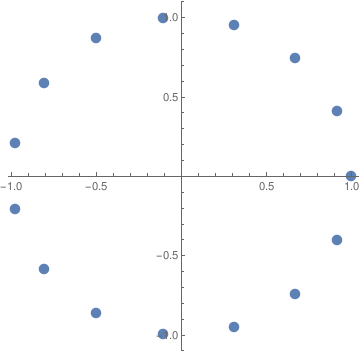Another code for all roots of 1: ShowNRoots[n_] := Module[{\[Omega] = Cos[2*Pi/n] + \[ImaginaryJ]*Sin[2*Pi/n]}, CPlot[Table[\[Omega]^k , { k, 0, n-1}]]]; ShowNRoots An elegant way of understanding the behavior of roots is to consider a root of z as z wanders through the complex plane $$\mathbb{C} .$$ We shall do this by just plotting either the real part or the imaginary part of the n-th root of z as z varies in a disc around the origin. In polar coordinates, we get a function \[ \mbox{Real part}(t, \theta ) = r^{1/n} \cos \left( \frac{\theta + t\,2\pi}{n} \right) , \qquad\mbox{or} \qquad \mbox{Imaginary part}(t, \theta ) = r^{1/n} \sin \left( \frac{\theta + t\,2\pi}{n} \right) , \quad t=0,1,\ldots , n-1.$
Let t vary from 0 to (2n-1)π to have all values of the root. These plots show how a multi-valued surface is generated. As one loops around the origin in units of 2π, one jumps from one root to the next.
ViewRootSurface[n_Integer, resolution_Integer] :=
ParametricPlot3D[{r*Cos[theta], r*Sin[theta], r^(1/n) * Cos[theta/n]},
{r, 0, 2}, {theta, 0, 2*n*Pi},
PlotPoints -> {resolution, resolution*n},
Boxed -> False, Axes ->False, AspectRatio -> 1, ViewPoint -> {-3, -3, 0}]
Here is the plot for a square root (n=2), showing the two-valued nature of the square-root function:
ViewRootSurface[2, 20]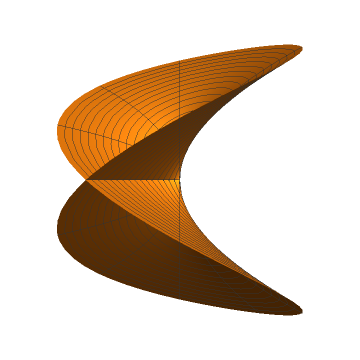A similar view of the logarithm function reveals how the function never joins up again. Here we plot the imaginary part, which is just the argument:
ViewLogSurface[n_Integer, resolution_Integer] :=
ParametricPlot3D[{r*Cos[theta], r*Sin[theta], theta},
{r, 0, 2}, {theta, 0, 2*n*Pi},
PlotPoints -> {resolution, resolution*n},
Boxed -> False, Axes ->False, AspectRatio -> 1/2, ViewPoint -> {-3, -2, 3}]
ViewLogSurface[2, 20]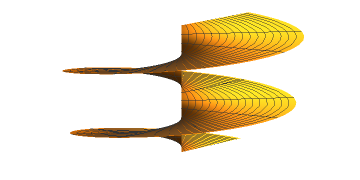CPlot[z_List] :=
Module[{r}, r = Map[{Re[#], Im[#]} &, z];
ListPlot[r, PlotStyle -> PointSize[0.1], AspectRatio ->1,
PlotRange -> {{-1.1, 1.1}, {-1.1, 1.1}},
PlotRegion -> {{0.05, 0.95}, {0.05, 0.95}}]]
We plot three cube roots of unity
omega = (-1 + I*Sqrt)/2
CPlot[{1, omega, omega^2}]